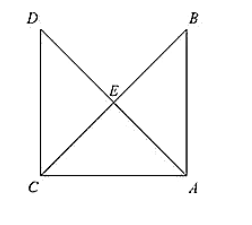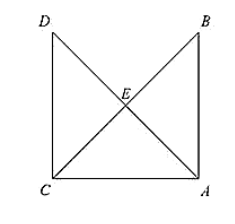# In figure, It is given that AB = CD and AD = BC. Prove that ΔADC ≅ ΔCBA.`
Question:

In figure, It is given that AB = CD and AD = BC. Prove that ΔADC ≅ ΔCBA.Solution:

Given that in the figure AB = CD and AD = BC.

We have to prove ΔADC ≅ ΔCBA

Now,

We have

AB = CD                     [Given]